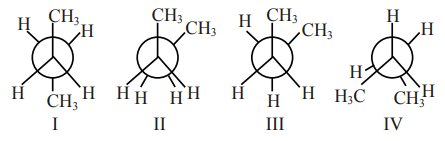# Arrange the following conformational isomers of n-butane in order of their increasing potential energy:`
Question:

Arrange the following conformational isomers of n-butane in order of their increasing potential energy:1. $\mathrm{II}<\mathrm{III}<\mathrm{IV}<\mathrm{I}$

2. $\mathrm{I}<\mathrm{IV}<\mathrm{III}<\mathrm{II}$

3. $\mathrm{II}<\mathrm{IV}<\mathrm{III}<\mathrm{I}$

4. $\mathrm{I}<\mathrm{III}<\mathrm{IV}<\mathrm{II}$

Correct Option: , 4

Solution:

More stable less potential energy.

Stability order : I > III > IV > II

So

Potential energy: II $>$ IV $>$ III $>$ I